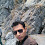## Multiple Choice Questions(MCQ) on Network Theorem

1. Kirchhoff s current law states that
(a) net current flow at the junction is positive
(b) Algebraic sum of the currents meeting at the junction is zero
(c) no current can leave the junction without some current entering it.
(d) total sum of currents meeting at the junction is zero

Ans: b

2. Kirchhoffs current law is applicable to only
(a) junction in a network
(b) closed loops in a network
(c) electric circuits
(d) electronic circuits

Ans: a

3. Thevenin resistance Rth is found
(a) by removing voltage sources along with their internal resistances
(b) by short-circuiting the given two terminals
(c) between any two ‘open’ terminals
(d) between same open terminals as for Eth

Ans: d

4. An ideal voltage source should have
(a) large value of e.m.f.
(b) small value of e.m.f.
(c) zero source resistance
(d) infinite source resistance

Ans: c

5. To determine the polarity of the voltage drop across a resistor, it is necessary to know
(a) value of current through the resistor
(b) direction of current through the resistor
(c) value of resistor
(d) e.m.fs. in the circuit

Ans: b

6. Which of the following is non-linear circuit parameter ?
(a) Inductance
(b) Condenser
(c) Wire wound resistor
(d) Transistor

Ans: a

7. For high efficiency of transfer of power, internal resistance of the source should be
(a) equal to the load resistance
(b) less than the load resistance
(c) more than the load resistance
(d) none of the above

Ans: a

8. The circuit whose properties are same in either direction is known as
(a) unilateral circuit
(b) bilateral circuit
(c) irreversible circuit
(d) reversible circuit

Ans: b

9. The circuit has resistors, capacitors and semi-conductor diodes. The circuit will be known as
(a) non-linear circuit
(b) linear circuit
(c) bilateral circuit
(d) none of the above

Ans: a

10. Which of the following is not a nonlinear element ?
(a) Gas diode
(b) Heater coil
(c) Tunnel diode
(d) Electric arc

Ans: b

11. Application of Norton’s theorem to a circuit yields
(a) equivalent current source and impedance in series
(b) equivalent current source and impedance in parallel
(c) equivalent impedance
(d) equivalent current source

Ans: b

12. Millman’s theorem yields
(a) equivalent resistance
(b) equivalent impedance
(c) equivalent voltage source
(d) equivalent voltage or current source

Ans: d

13. The superposition theorem is applicable to
(a) voltage only
(b) current “only
(c) both current and voltage
(d) current voltage and power

Ans: d

14. Between the branch voltages of a loop the Kirchhoff s voltage law imposes
(a) non-linear constraints
(b) linear constraints
(c) no constraints
(d) none of the above

Ans: b

15. While calculating Rth in Thevenin’s theorem and Norton equivalent

Ans: a

16. The number of independent equations to solve a network is equal to
(a) the number of chords
(b) the number of branches
(c) sum of the number of branches and chords
(d) sum of number of branches, chords and nodes

Ans: a

17. The superposition theorem requires as many circuits to be solved as there are
(a) sources, nodes and meshes
(b) sources and nodes
(c) sources
(d) nodes

Ans: c

Tags--
#MCQ on electrical engineering
#network theorems mcq.
Share:

1.2.Hi
One of the reasons why electronics field have big network because bof network theory

3.Question 11 , correct and is B

1.4.Ans of 13 is wrong. Superposition theorem cannot be used for power. So it is applicable for Voltage and current only.

1.Thanks for the suggestion